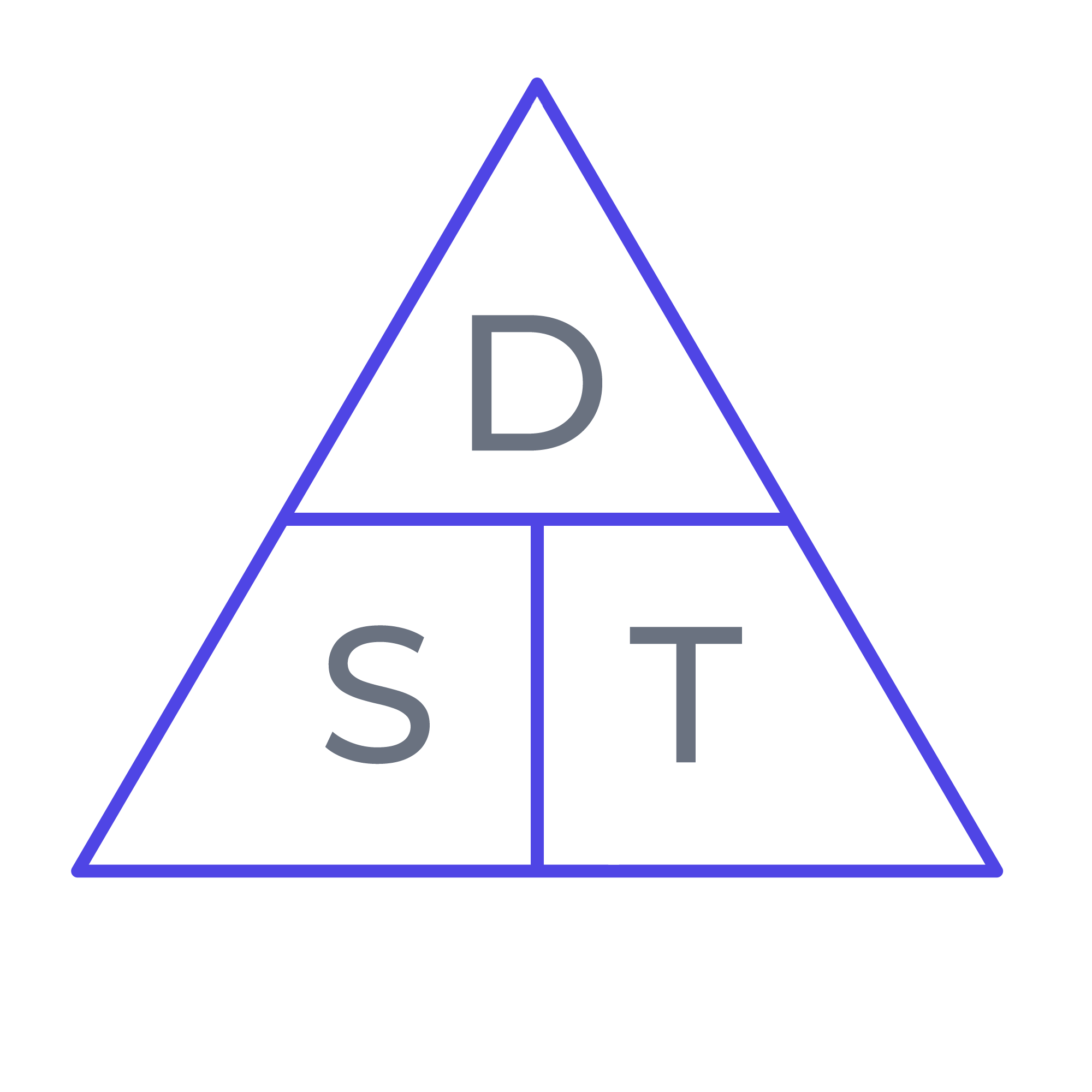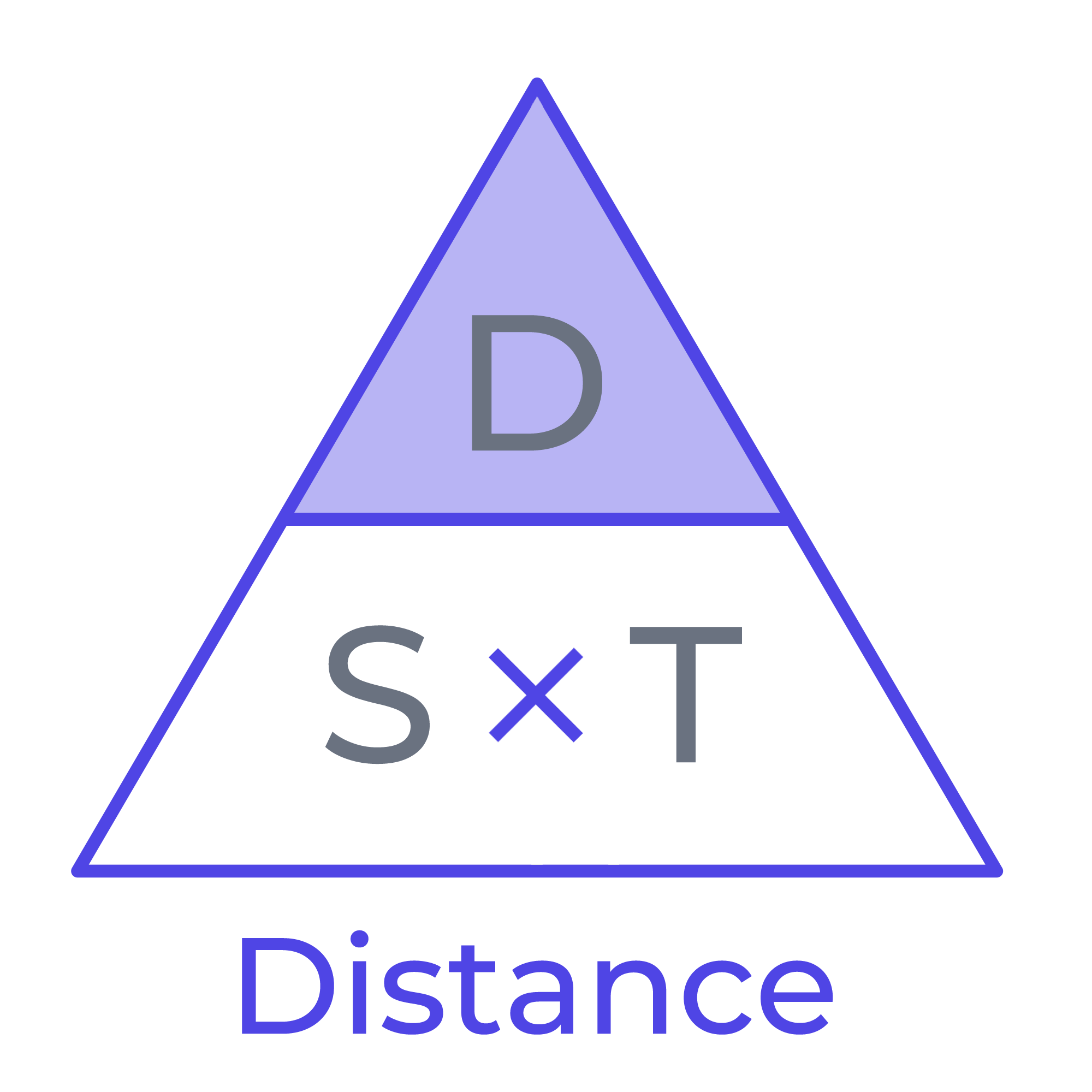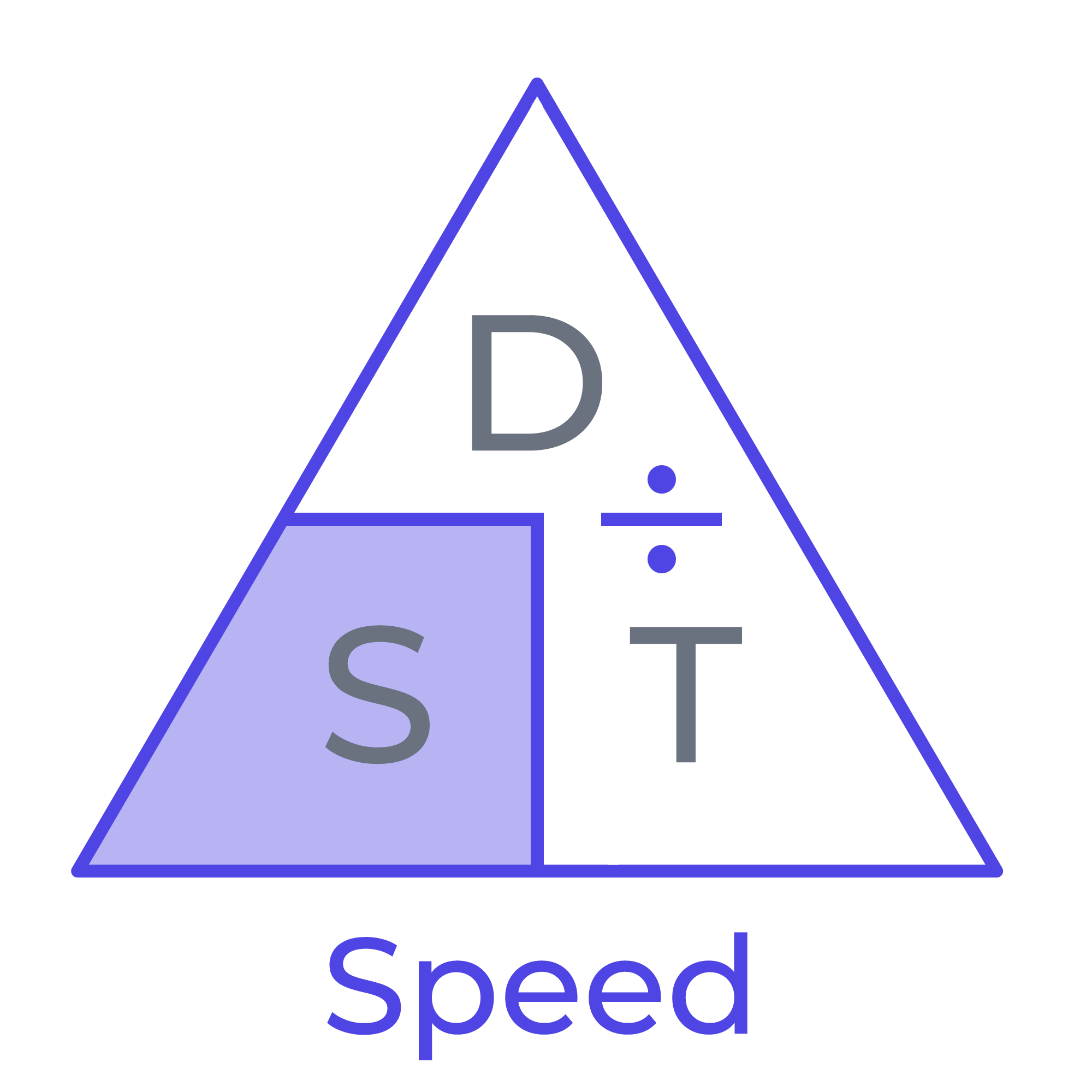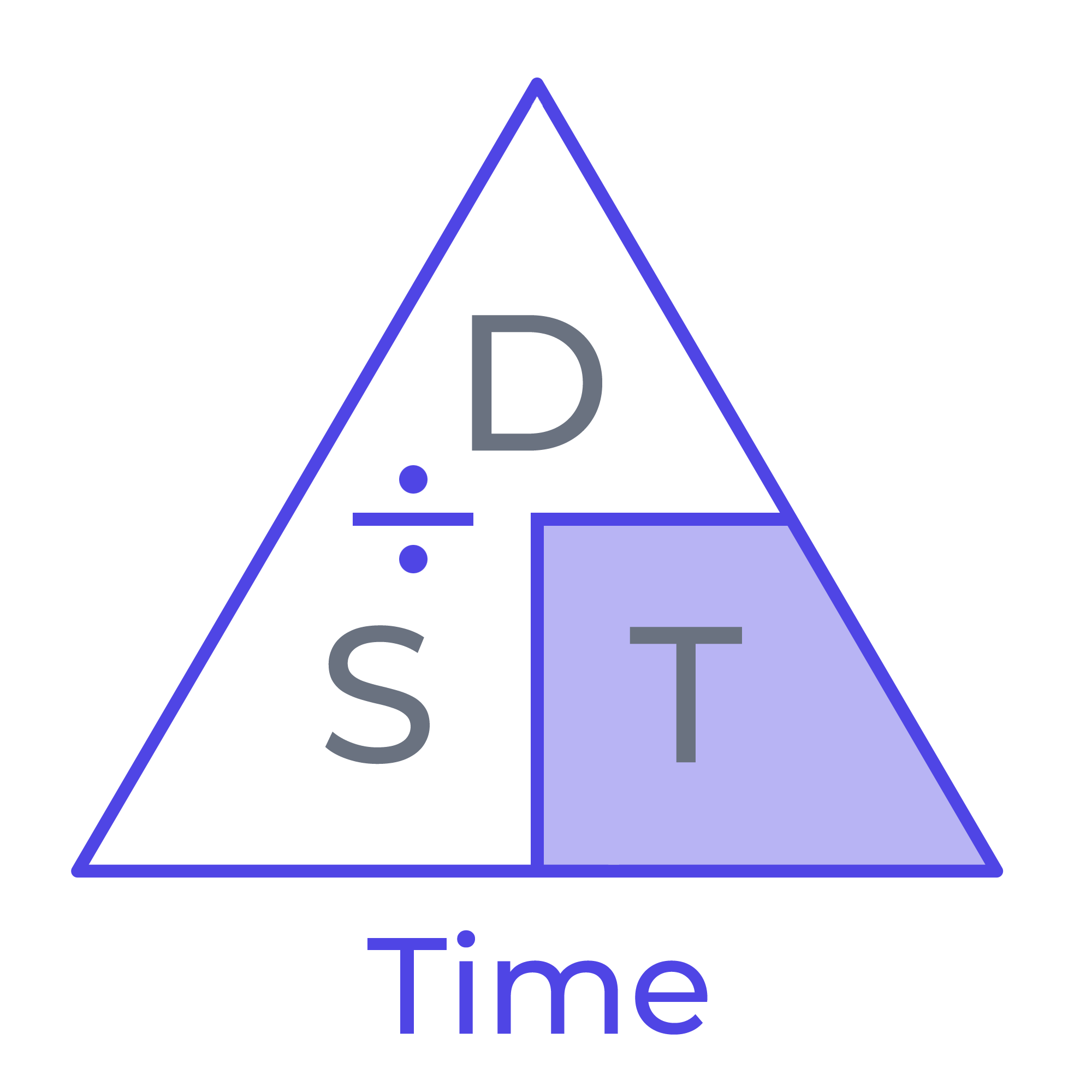Math Calculators
Speed Calculator

# Speed Calculator

The speed calculator helps find speed, distance, time using the formulas d=st, s=d/t, t=d/s. Easy to use and intuitive speed calculator.

280355.04km or 174133.57mi

There was an error with your calculation.Are you tired of the tedious task of manual calculations regarding speed, distance, and time? Pay attention to the Speed Distance Time Calculator. This powerful tool utilizes the fundamental formula d = st, making it easier to figure out these numbers precisely and accurately.

With the ability to determine distance, speed, and time through formulas such as d = st to calculate distance, s = d/t to calculate speed, and t = d/s to calculate time, this versatile time distance calculator can deduce an unknown value if two other values are known.

Furthermore, this distance speed calculator accepts time in various units, including seconds, minutes, hours, days, and years, and can handle a plethora of separators.

Speed distance and time calculator also allows calculating speed in multiple units, including miles per hour, kilometers per hour, meters per second, and many other speed measurement units, and distance in inches, feet, yards, miles, centimeters, meters, and kilometers.

Say goodbye to tedious calculations and hello to efficiency with the Speed Distance Time Calculator.

## DST triangle

Sometimes a so-called dst-triangle is used to represent the formula d = st more clearly. This representation helps you more easily remember what the formula for calculating distance, speed, and time looks like. And it also makes it easier to transform that formula to calculate one of these parameters. In the basic version, this triangle looks like this:If we don't know the distance, we can put the triangle like this and get a formula to calculate the distance based on speed and time:So the formula in this case would look like d = st.

If we don't know the speed, but need to calculate it based on distance and time data, we can represent the triangle in the following form and quickly recall the formula:So in this situation, we'll use the formula s = d/t.

And in the end, if we need to find the time, but have distance and speed information, we can use this version of the speed distance and time triangle:In a situation like this, we would apply the formula t = d/s to our calculations.

## Practical application

Calculating speed, distance, and time may seem like a dry and boring topic, but they are actually incredibly fascinating and useful concepts to understand. Whether you're trying to get somewhere on time or just trying to figure out how long it will take to get from point A to point B, understanding the relationship between speed, distance, and time is crucial.

The speed, distance, and time calculator is a helpful tool for physics students, engineers, and anyone who needs to make calculations related to speed, distance, and time. Even if you are not related to science, you can use time distance calculator in your everyday life. What simple daily calculations can it be useful for?

### Time Calculation

The formula used to calculate time is:

t = d/s

where t is time, d is distance, and s is speed.

In this equation, time equals distance divided by speed.

Let's look at an example of calculating time. We have quite an experienced runner who estimates their chances of running a marathon with good results. Our athlete wants to know how long it will take them to run a marathon (26.2 miles) at 9 miles per hour.

By the way, leading amateur marathon runners who can finish the marathon in less than three hours run 26.2 miles (42 kilometers) at an average speed of 8.8 miles per hour (or around 14.1 kilometers per hour). That's already a decent speed, considering that riding a bicycle may occur at about the same speed.

The runner can substitute the known values of distance (26.2 miles) and speed (9 miles per hour) using the formula t = d/s.

t = d/s = 26.2 / 9 = 2.91 hours

It will take 2.91 hours to run a marathon.

And the speed, distance, and time calculator will give us the result of the computation with staggering accuracy:

Time = 2.91111 hours = 2.91111 h = 02:54:40 = 2 hours, 54 minutes, and 40 seconds.

### Distance Calculation

We use the distance traveled formula

d = st

to determine the distance, where d stands for distance, s is speed, and t is time.

This formula is based on the principle that distance equals speed times time.

The following example will show us how to calculate distance. A driver plans a trip across the U.S. and wants to know how the distance he can cover in a certain time while moving at a certain speed. In this case, we can use our tool as a distance traveled calculator.

To begin, the driver tries to estimate how many miles they could cover if they drove at an average speed of 55 miles per hour for four hours without stopping.

Using the formula d = st, the driver can substitute the known values of speed (55 mph), and time (4 hours) to calculate the distance they will travel:

d = st = 55 × 4 = 220 miles

So, the distance of the trip will be 220 miles. This is a good distance to travel across the country. For example, the distance between New York City and Washington, D.C., is about 226 miles.

### Speed Calculation

To calculate speed, we use the speed formula

s = d/t

where s denotes speed, d is distance, and t is time. Here, the speed equals the distance divided by the time traveled. Applying this formula, you can use this calculator as an average speed calculator.

The following example will demonstrate the speed calculation. A plane leaves New York and is directed to London. These two cities are separated by a distance of 3,461 miles. The flight we are considering in this example takes 7 hours. How to calculate speed of the plane in miles per hour?

Using the formula s = d/t, we can substitute the known values of distance (3461 miles) and time (7 hours) to calculate the speed:

s = d/t = 3461 / 7 = 494.43 mph

So, the plane's average speed will be 494.43 mph, or around 494 mph.

If we are curious enough and decide to use a Speed Distance Time calculator to give us the results of these calculations in other units, it will provide us with even more comprehensive information. The aircraft's speed in this example is 0.137341 miles per second, 795.706 kilometers per hour, 221.029 meters per second.

## The History of Motion Calculation

The above equations seem simple enough to us. But scientists have spent a long time creating them and proving that they work. Ancient thinkers made serious contributions to the study of speed and distance. The Greek philosopher Aristotle was one of the first to systematically explore these topics.

Aristotle viewed time as a quantitative characteristic of motion and compared it to a number or measure of motion. Aristotle emphasized that time is not identical with motion, but is constantly connected with it. He viewed time as a flow, and each "now" as the end of the past and the beginning of the future. He viewed time as a thing and emphasized its measurement by means of numbers.

Aristotle's studies of the relationship of time, motion, and distance laid the foundation for future scientists and mathematicians. Greek astronomers such as Hipparchus and Ptolemy developed the field of astronomy, using mathematics to predict the motion of celestial bodies.

In the 17th century, the Italian scientist and mathematician Galileo Galilei made significant contributions to the study of motion and speed. He conducted observations and experiments with falling objects. This led him to suggest that the distance an object travels is directly proportional to the time it takes to travel that distance. He also suggested that the speed of an object is constant as long as the force acting on it is constant.

Galileo Galilei first proposed the concept of uniformly accelerated motion. He also studied the motion of pendulums and suggested that the length of a pendulum had nothing to do with how far it swung. Galileo Galilei's idea that distance and time are inversely related led to the equation d = st (distance = speed × time). It was Galileo Galilei who quantified speed in terms of distance and time.

The formula d = st (distance = speed × time) itself goes back to the work of French mathematician and physicist Pierre-Simon Laplace. He introduced the formula in 1799 in his book Celestial Mechanics. Laplace used it to calculate the distances of celestial bodies from Earth and to predict their future positions. This significant achievement in astronomy laid the foundation for celestial mechanics and the modern development of astrometry.

Incidentally, the formula d = st is a special case of the more general formula d = vt + 1/2at², where a stands for acceleration. This formula is an equation of motion of an object with constant acceleration, also known as the second equation of motion or kinematic equation of motion.

Its first part (vt) represents the distance traveled due to initial velocity, and its second part (1/2at²) represents the distance traveled due to acceleration.

## Conclusion

The Speed, Distance and Time calculator is a valuable aid for those who need accurate and reliable calculations related to speed, distance and time. Whether you are a physics student, an engineer, or just someone who needs a quick calculation, this tool will make your calculations easy and accurate.

With its versatile handling of different units, use of different delimiters and the ability to get results in different units, the speed calculator comes in handy for a wide variety of tasks.

Say goodbye to tedious manual recalculations and arm yourself with accuracy and precision with the speed time and distance calculator!